## How to - Get PROC MEANS Output By Using PROC TABULATE Procedure(i.e Mean and Std Dev)

Hello everyone,

I try to create PROC MEANS output by using PROC TABULATE. Actually, It is little bit complicated. I also do some additonal step on Excel after I got PROC MEANS output. But I think PROC TABULATE can provides me to reach my desired report output. I have a sample code as below and my desired output also as below. Is it possible to create my desired output based on this sample data set?

``````Data Have;
Length Target 8 Numeric1 8 Numeric2 8 Numeric3 8 Numeric4 8;
Infile Datalines Missover;
Input Target Numeric1 Numeric2 Numeric3 Numeric4;
Datalines;
0 0.2 0.4 0.1 0.0
1 0.3 0.5 0.2 0.2
0 0.4 0.8 0.9 0.1
1 0.6 0.9 0.0 0.2
1 0.2 0.1 0.4 0.3
0 0.3 0.1 0.3 0.2
1 0.7 0.1 0.1 0.4
1 0.1 0.8 0.3 0.3
;
Run;

PROC MEANS DATA=Have
FW=12 PRINTALLTYPES CHARTYPE NWAY VARDEF=DF MEAN STD N;
VAR Numeric1 Numeric2 Numeric3 Numeric4;
CLASS Target /   ORDER=UNFORMATTED ASCENDING;

RUN;

ODS OUTPUT Summary=DS_1(Rename=(MEAN=_MEAN STDDEV=_STDDEV N=_N)DROP=_:);
PROC MEANS DATA=Have MEAN STD N STACKODSOUTPUT;
VAR Numeric1 Numeric2 Numeric3 Numeric4;
CLASS Target / ORDER=UNFORMATTED ASCENDING;
RUN;``````
``````PROC TABULATE DATA=HAVE;
VAR Target;
CLASS  Numeric1 Numeric2 Numeric3 Numeric4;
TABLE (Numeric1*ALL) ALL (Numeric2*ALL) ALL (Numeric3*ALL) ALL (Numeric4*ALL) ALL,
Target*Mean Target*N Target*StdDev Target*All;
RUN;
``````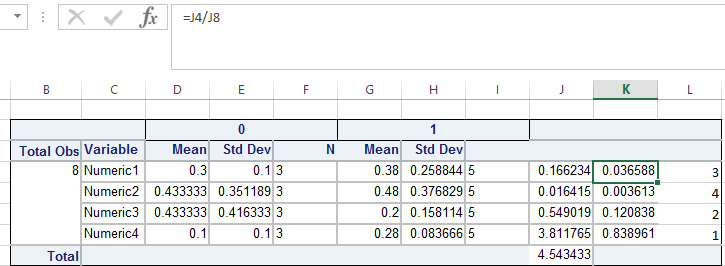Thank you

1 ACCEPTED SOLUTION

Accepted Solutions

## Re: How to - Get PROC MEANS Output By Using PROC TABULATE Procedure(i.e Mean and Std Dev)

Hi:

Hi,
And, in addition to BallardW's note about the CLASS and VAR usage, with PROC TABULATE, you can only request a statistic in 1 dimension. In your TABULATE example, you have statistics requested in multiple dimensions (row and col) and you should be seeing something like this in the LOG, even if you fix the CLASS and VAR problem:
ERROR: Statistic other than N was requested without analysis variable in the following nesting : All * Target *  Mean.
...or a whole bunch of messages like this for every statistic.  If you have access to our Report Writing course notes, please look in the book in the TABULATE chapter for an explanation of how statistics can only be requested in one dimension, or look in the documentation examples -- they will always show the statistic in one dimension or the other, not both.

Note that in BallardW's example, the requested statistics are ONLY in the column dimension. You can approximate what you get from PROC MEANS using code like this:

``````title '1) Your original PROC MEANS';
PROC MEANS DATA=Have
FW=12 PRINTALLTYPES CHARTYPE NWAY VARDEF=DF MEAN STD N;
VAR Numeric1 Numeric2 Numeric3 Numeric4;
CLASS Target /   ORDER=UNFORMATTED ASCENDING;

RUN;

proc tabulate data=have;
title '2) Alternate Suggestion with TABULATE';
class Target;
var  Numeric1 Numeric2 Numeric3 Numeric4;
TABLE Target *(Numeric1 Numeric2 Numeric3 Numeric4) ,
(Mean*f=9.4 StdDev*f=9.4 N );
RUN;
``````

produces this: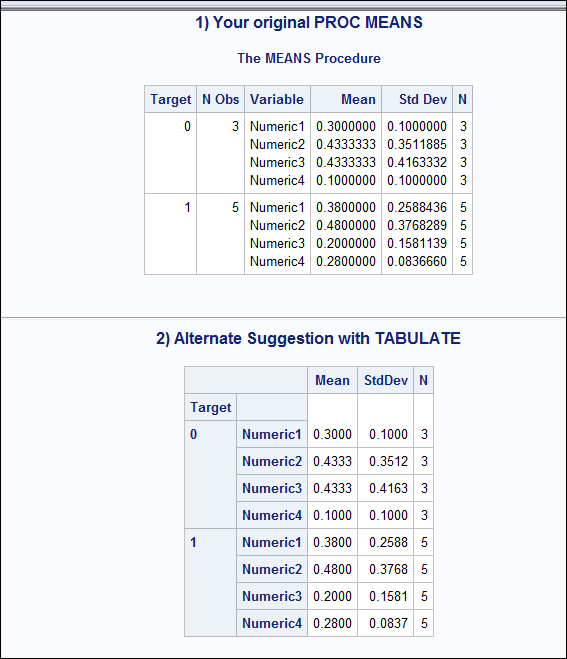You can change the format to change the number of decimal places. I don't see where you need the ALL to replicate what you get from PROC MEANS.

And, if you restructure the data, you can also replicate the PROC MEANS output with PROC REPORT, as shown here (which might be useful if you need to add your own calculations):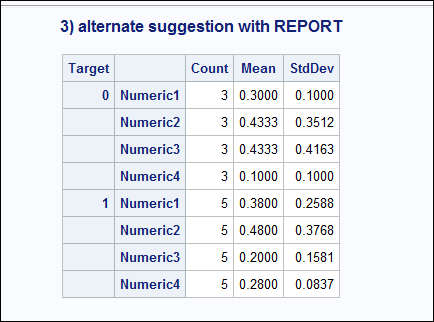I didn't both to use SPANROWS to make it look the same as TABULATE, but that is simple enough to do in the REPORT code:

``````
data new_have;
set have;
varname = 'Numeric1';
val = numeric1;
output;
varname = 'Numeric2';
val = numeric2;
output;
varname = 'Numeric3';
val = numeric3;
output;
varname = 'Numeric4';
val = numeric4;
output;
run;

proc report data=new_have;
title '3) alternate suggestion with REPORT';
column target varname val val=valmn val=valstdv;
define target / group style(column)=Header 'Target';
define varname / group style(column)=Header ' ';

define val / n 'Count';
define valmn / mean 'Mean' f=9.4;
define valstdv / stddev 'StdDev' f=9.4;
run;``````

Hope this helps.

cynthia

12 REPLIES 12

## Re: How to - Get PROC MEANS Output By Using PROC TABULATE Procedure(i.e Mean and Std Dev)

You have reversed the rols of Class and Var variables. The Class varaibles are grouping and the Var variables are ones that you request statistics for. This gets what you want for the part under the Target variable:

``````proc tabulate data=have;
class Target;
var  Numeric1 Numeric2 Numeric3 Numeric4;
TABLE Numeric1 Numeric2 Numeric3 Numeric4 ,
(Target )*(Mean*f=best6. StdDev*f=best7. N );
RUN;``````

I have no idea what any of that stuff in columns J, K and L might be but I suspect that will not come from Proc tabulate.

The formats, f=best , above are for demonstration. You can get more or fewer decimals by changing display length.

## Re: How to - Get PROC MEANS Output By Using PROC TABULATE Procedure(i.e Mean and Std Dev)

Hello @ballardw,

Thank you very much for your quick response.

Yes, it is not possible to get this report being SAS data set 😞  I need to do the following formula over your report output.

Desired=2*((Mean0-Mean1)**2)/(StdDev0-StdDev1))

Desired2=Desired/Sum(Desired)

So it is not possible to perform the foregoing formulas on your report output, do you have a workaround idea to do this?

Thank you,

## Re: How to - Get PROC MEANS Output By Using PROC TABULATE Procedure(i.e Mean and Std Dev)

Proc Tabulate will not do calculations involving result columns. It is not designed that way.

Proc report will allow doing column calculations though. I don't us Proc Report much so it'll take longer, or someone else may get a result first.

Note that column dependent calculations are very dependent on explicit column numbers in proc report. If the number of columns of your target variable changes then the calculation code needs to be redone.

## Re: How to - Get PROC MEANS Output By Using PROC TABULATE Procedure(i.e Mean and Std Dev)

Hi @ballardw,

You are totally right.

I know the PROC TABULATE don't calculate involving result columns but I wanted to ask that can we go over a long method. I mean maybe we can do this by using 6 Data Steps, is it possible?

Thank you,

## Re: How to - Get PROC MEANS Output By Using PROC TABULATE Procedure(i.e Mean and Std Dev)

Hi:

Hi,
And, in addition to BallardW's note about the CLASS and VAR usage, with PROC TABULATE, you can only request a statistic in 1 dimension. In your TABULATE example, you have statistics requested in multiple dimensions (row and col) and you should be seeing something like this in the LOG, even if you fix the CLASS and VAR problem:
ERROR: Statistic other than N was requested without analysis variable in the following nesting : All * Target *  Mean.
...or a whole bunch of messages like this for every statistic.  If you have access to our Report Writing course notes, please look in the book in the TABULATE chapter for an explanation of how statistics can only be requested in one dimension, or look in the documentation examples -- they will always show the statistic in one dimension or the other, not both.

Note that in BallardW's example, the requested statistics are ONLY in the column dimension. You can approximate what you get from PROC MEANS using code like this:

``````title '1) Your original PROC MEANS';
PROC MEANS DATA=Have
FW=12 PRINTALLTYPES CHARTYPE NWAY VARDEF=DF MEAN STD N;
VAR Numeric1 Numeric2 Numeric3 Numeric4;
CLASS Target /   ORDER=UNFORMATTED ASCENDING;

RUN;

proc tabulate data=have;
title '2) Alternate Suggestion with TABULATE';
class Target;
var  Numeric1 Numeric2 Numeric3 Numeric4;
TABLE Target *(Numeric1 Numeric2 Numeric3 Numeric4) ,
(Mean*f=9.4 StdDev*f=9.4 N );
RUN;
``````

produces this:You can change the format to change the number of decimal places. I don't see where you need the ALL to replicate what you get from PROC MEANS.

And, if you restructure the data, you can also replicate the PROC MEANS output with PROC REPORT, as shown here (which might be useful if you need to add your own calculations):I didn't both to use SPANROWS to make it look the same as TABULATE, but that is simple enough to do in the REPORT code:

``````
data new_have;
set have;
varname = 'Numeric1';
val = numeric1;
output;
varname = 'Numeric2';
val = numeric2;
output;
varname = 'Numeric3';
val = numeric3;
output;
varname = 'Numeric4';
val = numeric4;
output;
run;

proc report data=new_have;
title '3) alternate suggestion with REPORT';
column target varname val val=valmn val=valstdv;
define target / group style(column)=Header 'Target';
define varname / group style(column)=Header ' ';

define val / n 'Count';
define valmn / mean 'Mean' f=9.4;
define valstdv / stddev 'StdDev' f=9.4;
run;``````

Hope this helps.

cynthia

## Re: How to - Get PROC MEANS Output By Using PROC TABULATE Procedure(i.e Mean and Std Dev)

Hello @Cynthia_sas,

Thank your explanations about the requested statistics for PROC TABULATE, I will try to be more careful. I will investigate the books which you mentioned them.

Also thank you for the PROC REPORT example,

But I want to see "0" and "1" step by step, instead of one under the other. If I get the following report in the PROC REPORT, I can do my calculations.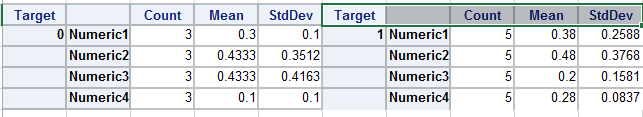If get the foregoing table by using PROC REPORT then I can maybe do some calculations over this table by using Compute statement.

Actually, @ballardw's sample provides the foregoing table but because of his sample is created by using PROC TABULATE, I cannot use Compute statement.

``````proc tabulate data=have;
class Target;
var  Numeric1 Numeric2 Numeric3 Numeric4;
TABLE Numeric1 Numeric2 Numeric3 Numeric4 ,
(Target )*(Mean*f=best6. StdDev*f=best7. N );
RUN;``````

As you always tell me, it is impossible to get PROC TABULATE output being data set. But to reach my desired output, I need two additional columns.

I understood PROC TABULATE doesn't provide my desired output.

Maybe you gave tip to me in your PROC REPORT example, but I'm not sure whether I can proceed over your PROC REPORT statement to reach my aim.

I need the following calculations ->

Desired=2*((Mean0-Mean1)**2)/(StdDev0-StdDev1))

Desired2=Desired/Sum(Desired)

In my foregoing post, I tried to ask to @ballardw, is it possible to create the output by using DATA STEPs, even it will take 8-9 Data Steps and affect the performance inefficient.

I don't know, I'm just asking, if it is not possible, I'm okay. Or if there is a method to show the same report in a different way, I'm glad to listen you.

Thank you,

## Re: How to - Get PROC MEANS Output By Using PROC TABULATE Procedure(i.e Mean and Std Dev)

Hi:

If you need additional calculations, then your choices are the PROC REPORT route, but you'll have to restructure your data to get what you want from PROC REPORT. It does not usually create tables such as you show ...generally with PROC REPORT, your TARGET variable would be ACROSS and your Numeric1, etc variables would be going down the rows as GROUP variables. But they are usually on the left-most row area of the report and not on the left and also in the middle before each group. And, with PROC REPORT, if you used ACROSS variables, then these calculations

Desired=2*((Mean0-Mean1)**2)/(StdDev0-StdDev1))

Desired2=Desired/Sum(Desired)

would be hard to redo because instead of meaningful variable names, you'd have to switch to absolute column numbers for the formulas.

There are probably ways to do what you want, but the PROC REPORT would get complex. Possibly pre-summarizing, getting your statistics and then doing your calculations is the way to go. It depends on what you're comfortable doing and what the customer is comfortable having to maintain.

I suppose if you pre-summarized your data, then you could also investigate the Report Writing Interface -- but that works best in PDF and depending on your destination of choice, might not work as well in RTF or TAGSETS.EXCELXP, for example.

cynthia

## Re: How to - Get PROC MEANS Output By Using PROC TABULATE Procedure(i.e Mean and Std Dev)

Hello @Cynthia_sas,

Thank you for the information and your direction.

I think I can reach my aim by using your method. At this point, I have two questions to you;

1st- You did the following step before you create the report

``````data new_have;
set have;
varname = 'Numeric1';
val = numeric1;
output;
varname = 'Numeric2';
val = numeric2;
output;
varname = 'Numeric3';
val = numeric3;
output;
varname = 'Numeric4';
val = numeric4;
output;
run;``````

This is very useful but what if the variable number is more than 4 or less than 4, how can I provide dynamic structure for this step or similar method to provide my desired dynamic structure? Can you help me?

2nd->

The following code enable to do some calculations but I want to do some calculations over computed columns, is it possible to do this in PROC REPORT?

``````Proc Report data=new_have;
title 'Alternate Suggestion with REPORT';
column varname Target ,(val=valmn val=valstdv val) Variable1 Variable2  Variable3 Variable4;
define varname / group style(column)=Header 'Name';
define Target / across style(column)=Header 'Target';
define valmn / mean 'Mean' f=9.4;
define valstdv / stddev 'Standard Deviation' f=9.4;
define val / n 'N';
define Variable1 / 'Variable1' computed ;
define Variable2 / 'Variable2' computed ;
define Variable3 / 'Variable3' computed ;
define Variable4 / 'Variable4' computed ;
compute Variable1 ;
Variable1 =2*(((_c2_-_c5_)**2)/((_c3_)**2+(_c6_)**2));
endcomp;

compute Variable2;
Variable2=_c8_/(Sum(_c8_));
endcomp;

/*	  compute Variable3;*/
/*        Variable3=(Sum(_c8_);*/
/*     endcomp;*/
/**/
/*	 compute Variable3;*/
/*        Variable3=_c10_/(Sum(_c10_);*/
/*     endcomp;*/
run;``````

Thank you,

## Re: How to - Get PROC MEANS Output By Using PROC TABULATE Procedure(i.e Mean and Std Dev)

I try to implement your greate macro sample but I get some errors

``````%Let Variable=Numeric1 Numeric2 Numeric3 Numeric4;
%Let Count=%Sysfunc(CountW(&Variable));

%Put &Variable;
%Put &Count;

%Macro MakeVar;

%If &Count GT 0 %Then %Do;
%Do i = 1 %to &Count;
%Let Var&i = %str(varname=") %Scan(&Variable,&i)%str("; val=) %Scan(&Variable,&i) %str(; output;);
%Put Var&i= &&Var&i;
%End;
%End;
%Else %Do;
%Put ERROR: No value for Variable specified: &Variable;
%End;

%Mend  MakeVar;

%MakeVar;``````

Var1= varname=") "; val= Numeric1 ; output;
ERROR: Macro function %SCAN has too few arguments.

It doesn't resolve the macro variable because of double-quote("). But I'm using %str, shouldn't it help us to mask the values?

Thank you

## Re: How to - Get PROC MEANS Output By Using PROC TABULATE Procedure(i.e Mean and Std Dev)

I would do something a bit differently. I assume you are trying to dynamically generate the "restructured" dataset. You always have to keep in mind the program you need to generate. The Macro facility does NOT do any processing for you. It ONLY generates code. It is like a big, fat, dumb typewriter that only knows how to type code.

This is why when we teach the macro class we recommend that you start with a working SAS program and work backwards from the program that works and does what you want to "macro-izing" the code. I will post an example in a bit, after I find one.
cynthia

## Re: How to - Get PROC MEANS Output By Using PROC TABULATE Procedure(i.e Mean and Std Dev)

Hi:

Consider this macro definitioni program that, when invoked, generates the entire data step program to restructure the HAVE data from your original posting.

Here's the Macro program definition:

``````%Macro MakeVar;

data new_have;
set have;

%If &Count GT 0 %Then %Do;
%Do i = 1 %to &Count;
%Let Var&i = %Scan(&Variable,&i,%str( ));
%Put Var&i= &&Var&i;
varname = "&&var&i";
val = &&var&i;
output;
%End;
%End;
%Else %Do;
%Put ERROR: No value for Variable specified: &Variable;
%End;
run;
%Mend  MakeVar;``````

There is no need to put the %LET or %PUT before the macro definition, because they are not needed until the macro is invoked. So invoking with 4 values for &VARIABLE results in this: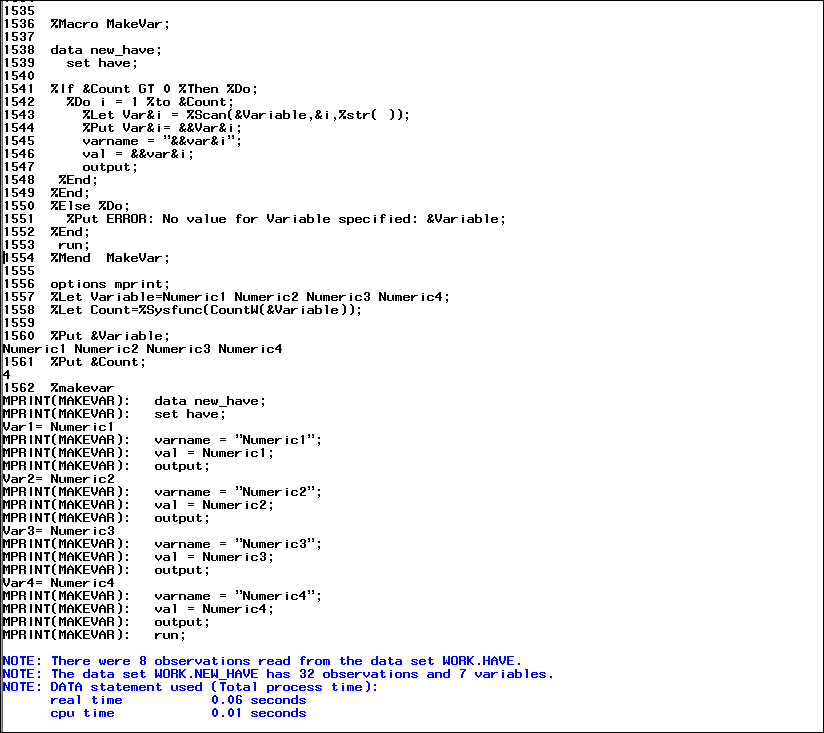and invoking with 5 values for &VARIABLE results in this: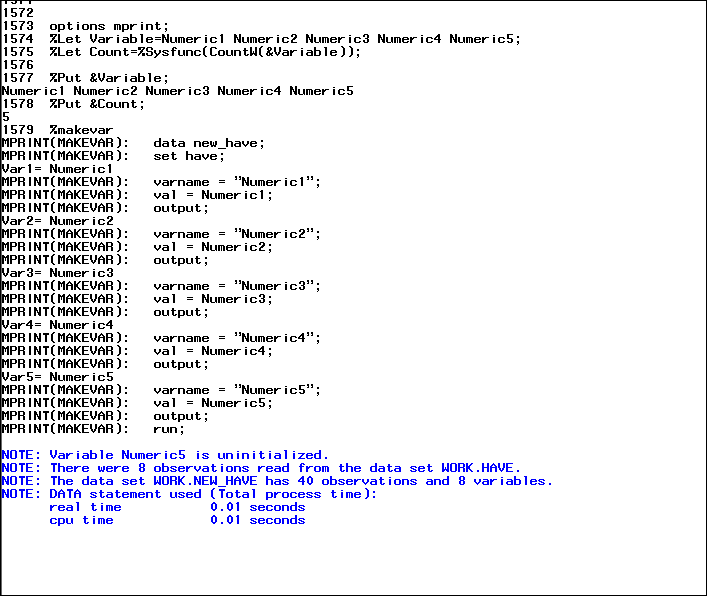Notice how the %LET and the %PUT are placed immediately BEFORE the macro definition is invoked. I also turned on the MPRINT option so you could see exactly what program was being typed for me by the Macro Processor.

This is fundamental to how the Macro Language processor operates. A macro program ONLY generates code -- by the time your code gets to the SAS compliler, there are NOT any %IF statements or %DO statements left -- all that's left to be compiled and executed is the generated code. You only needed to use %SCAN to extract the one token you needed and then use that token to build 2 different assignment statements. If you remember that the macro processor is only typing code then you can have whole programs, whole statements or parts of statements under the control of macro logic.

cynthia

## Re: How to - Get PROC MEANS Output By Using PROC TABULATE Procedure(i.e Mean and Std Dev)

Thank you very much for your invaluable sample. I sometimes forget to use MPRINT option but it is useful option, when we use the Macro processor.

I also know that there is no need to put the %LET or %PUT before the macro definition,but I will try to be careful, when write macro codes.

This is the dynamic structure which I talked in my previous posts., now, I have two meaningful macro samples to build dynamic structures. It is not easy to find this kind of codes 🙂  Thank you very much.

Discussion stats
• 12 replies
• 3151 views
• 3 likes
• 3 in conversation